## Eduwhere's Concept: Heron's Formula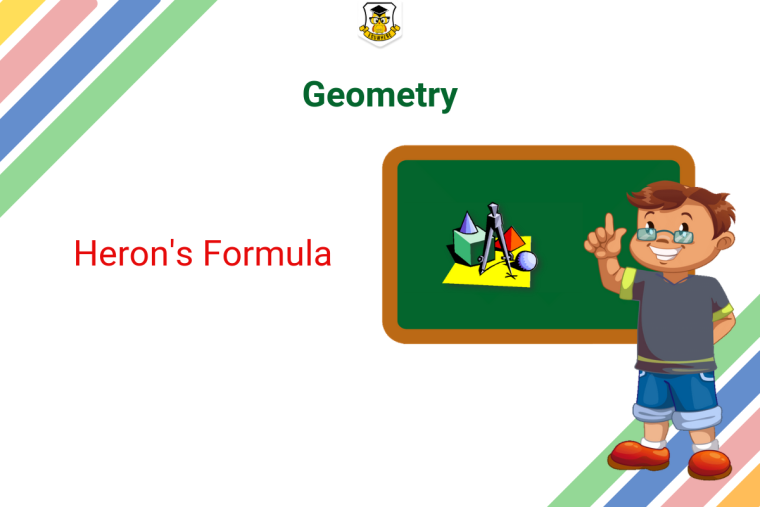In order to determine the area of a triangle, Heron’s formula in geometry. The area of a triangle can be calculated if the 3 sides of a triangle are known. For this formula, you need to know the length of all 3 sides of the triangle. This area formula makes the calculation of the area of a triangle by eliminating the use of angles and the need to calculate the height of the triangle.

Prepare for your exams with Eduwhere

Triangles

Let’s learn about all the three types of triangles now. The sum of all the angles of a triangle is 180°.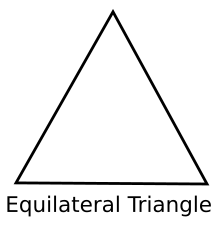Equilateral Triangle: A triangle with all sides of equal length and all angles of 60° is an equilateral triangle.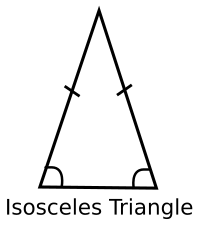Isosceles Triangle: A triangle with any 2 sides equal in length is called an isosceles triangle.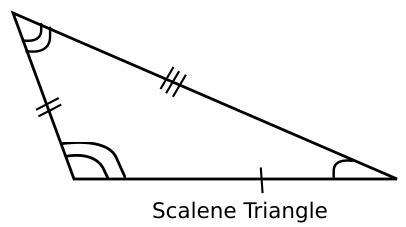Scalene Triangle: A triangle with no side equal in length to the other is called a scalene triangle.

Now that we know what triangles are, let’s take a look at Heron’s formula.

Heron’s Formula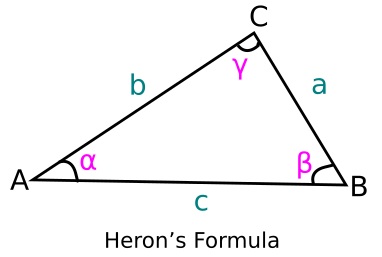Let’s consider the three sides of a triangle are ‘a’, ‘b’, ‘c’ units. We calculate the perimeter of the triangle as P = a + b + c. Hence, the semi perimeter of the triangle can be calculated as

s = P/2 = (a+b+c)/2

According to Heron’s formula, the triangle’s area formula is

√[s(s-a)(s-b)(s-c)]

Please note, the above area of triangle formula is applicable for all triangles irrespective of the lengths of their sides.

For more such informative updates and general knowledge articles, follow Eduwhere Community.

Eduwhere provides online mock tests for examinations like CAT, MAT, XAT, SNAP, CMAT, CLAT, SBI PO, SBI Clerk, SSC CGL, SSC CHSL, CTET, IBPS PO, IBPS Clerk, AFCAT and others.

With the Eduwhere Edge pass available for 99/- for 1 month and 199/- for 3 months, you can get complete access to all the mock tests available with us.

Prepare with Eduwhere Edge

Very Well Explained Thank You Eduwhere Community

3

3509